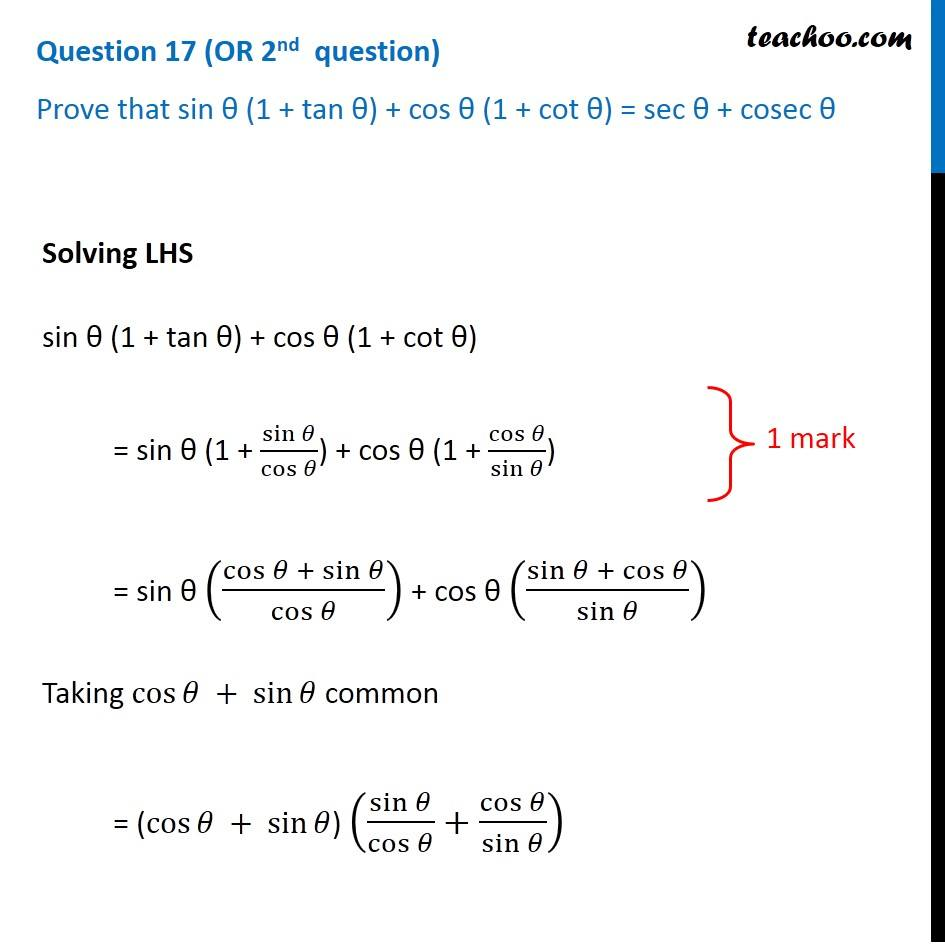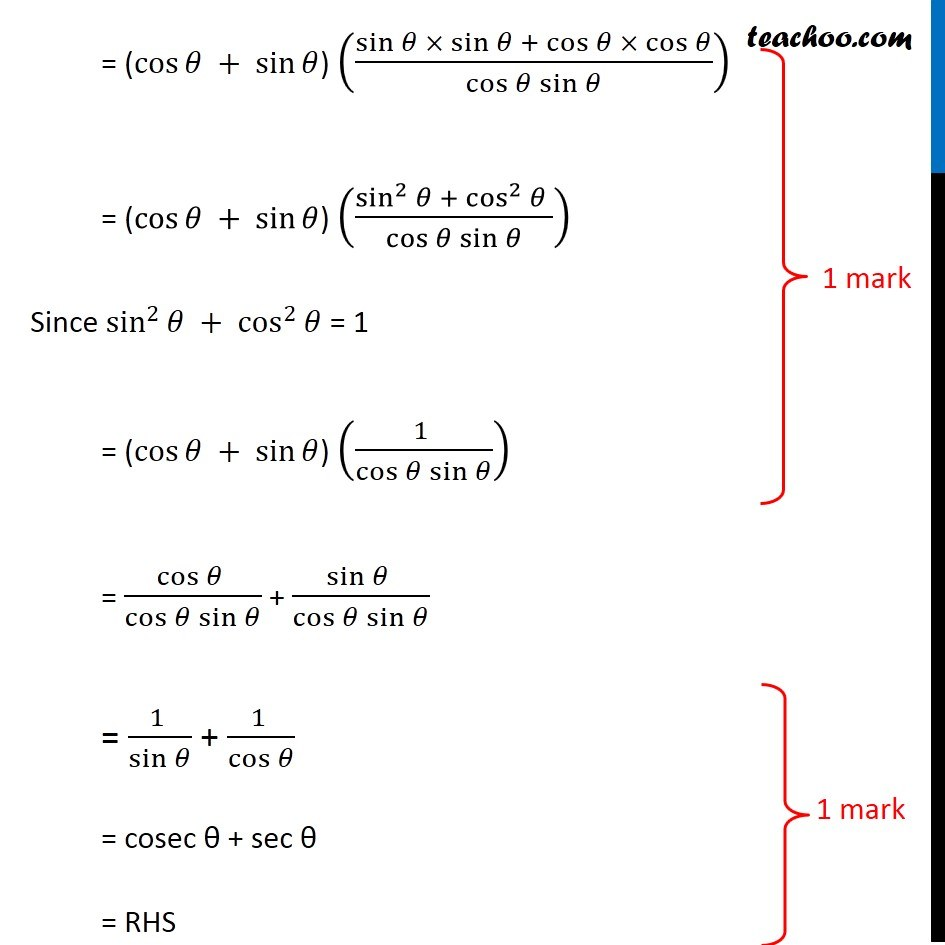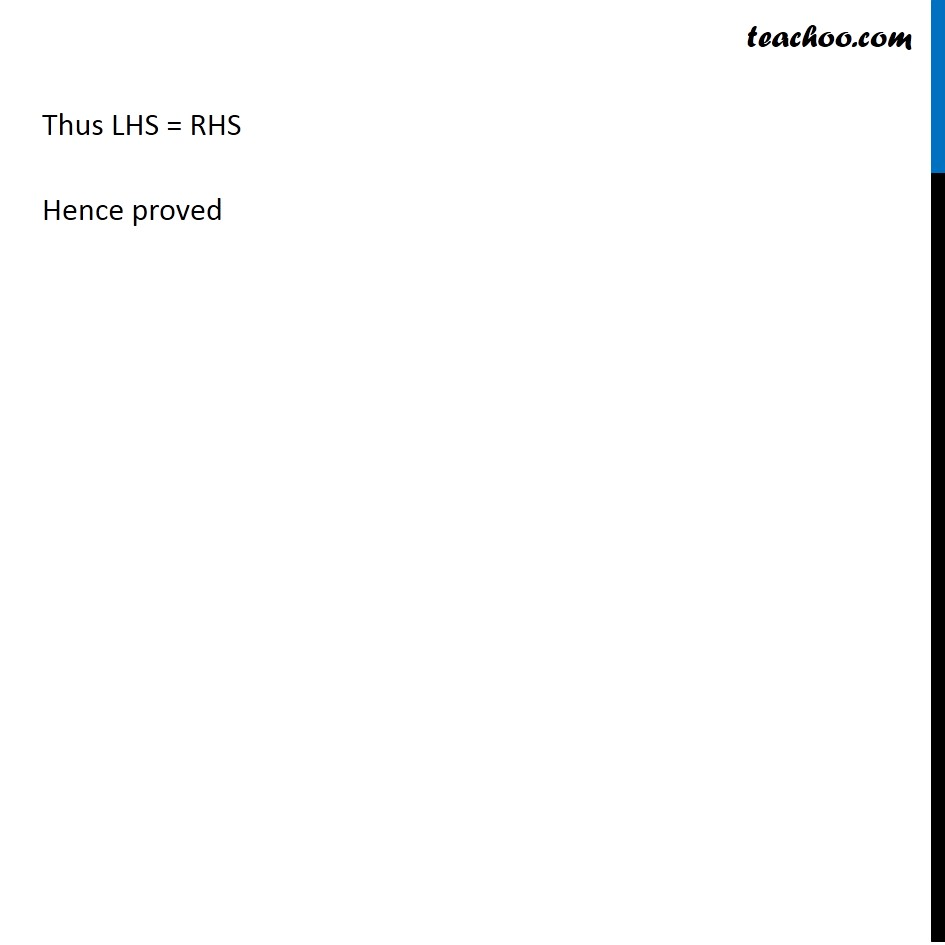Question 17 (OR 2 nd   question)

Prove that sin θ (1 + tan θ) + cos θ (1 + cot θ) = sec θ + cosec θ1. Class 10
2. Solutions of Sample Papers for Class 10 Boards
3. CBSE Class 10 Sample Paper for 2019 Boards

Transcript

Question 17 (OR 2nd question) Prove that sin θ (1 + tan θ) + cos θ (1 + cot θ) = sec θ + cosec θ Solving LHS sin θ (1 + tan θ) + cos θ (1 + cot θ) = sin θ (1 + sin⁡𝜃/cos⁡𝜃 ) + cos θ (1 + cos⁡𝜃/sin⁡𝜃 ) = sin θ ((cos⁡𝜃 + sin⁡𝜃)/cos⁡𝜃 ) + cos θ ((sin⁡𝜃 + cos⁡𝜃)/sin⁡𝜃 ) Taking cos⁡𝜃 + sin⁡𝜃 common = (cos⁡𝜃 + sin⁡𝜃) (sin⁡𝜃/cos⁡𝜃 +cos⁡𝜃/sin⁡𝜃 ) = (cos⁡𝜃 + sin⁡𝜃) ((sin⁡𝜃 × sin⁡𝜃 + cos⁡𝜃 × cos⁡𝜃)/(cos⁡𝜃 sin⁡𝜃 )) = (cos⁡𝜃 + sin⁡𝜃) ((sin^2⁡𝜃 + cos^2⁡𝜃 )/(cos⁡𝜃 sin⁡𝜃 )) Since sin^2⁡𝜃 + cos^2⁡𝜃 = 1 = (cos⁡𝜃 + sin⁡𝜃) ((1 )/(cos⁡𝜃 sin⁡𝜃 )) = (cos⁡𝜃 )/(cos⁡𝜃 sin⁡𝜃 ) + (sin⁡𝜃 )/(cos⁡𝜃 sin⁡𝜃 ) = (1 )/sin⁡𝜃 + (1 )/cos⁡𝜃 = cosec θ + sec θ = RHS Thus LHS = RHS Hence proved

CBSE Class 10 Sample Paper for 2019 Boards

Class 10
Solutions of Sample Papers for Class 10 Boards